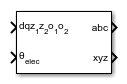# Inverse Decoupled Transform (Six-Phase)

Implement dqz1z2o1o2 to abc and xyz transform

• Library:
• Simscape / Electrical / Control / Mathematical Transforms

•## Description

The Inverse Decoupled Transform (Six-Phase) block converts the direct, quadrature, z1, z2, o1, and o2 components in a rotating reference frame to the components of a six-phase system in the abc and xyz reference frame. The three-phase systems abc and xyz are spatially shifted by 30 electrical degrees.

You can configure the block to align the phase a-axis of the five-phase system to either the d-axis or q-axis of the rotating reference frame at time t = 0.

θ = ωt + Offset is the angle between the a and q axes for the q-axis alignment or the angle between the a and d axes for the d-axis alignment, where:

• ω is the rotational speed of the rotational reference frame.

• t is the time, in s, from the initial alignment.

• Offset=\begin{array}{c}0,\text{\hspace{0.17em}}\text{\hspace{0.17em}}\text{\hspace{0.17em}}\text{\hspace{0.17em}}\text{\hspace{0.17em}}d-axis\text{\hspace{0.17em}}alignment\\ -\frac{\pi }{2},\text{\hspace{0.17em}}\text{\hspace{0.17em}}\text{\hspace{0.17em}}\text{\hspace{0.17em}}q-axis\text{\hspace{0.17em}}alignment\end{array}

### Equations

The Inverse Decoupled Transform (Six-Phase) block implements the transform as

`$\left[\begin{array}{c}a\\ b\\ c\\ x\\ y\\ z\end{array}\right]=\left[\begin{array}{cccccc}\mathrm{cos}\theta & -\mathrm{sin}\theta & 1& 0& 1& 0\\ \mathrm{cos}\left(\theta -\frac{2\pi }{3}\right)& -\mathrm{sin}\left(\theta -\frac{2\pi }{3}\right)& -\frac{1}{2}& -\frac{\sqrt{3}}{2}& 1& 0\\ \mathrm{cos}\left(\theta +\frac{2\pi }{3}\right)& -\mathrm{sin}\left(\theta +\frac{2\pi }{3}\right)& -\frac{1}{2}& \frac{\sqrt{3}}{2}& 1& 0\\ \mathrm{cos}\left(\theta -\frac{\pi }{6}\right)& -\mathrm{sin}\left(\theta -\frac{\pi }{6}\right)& -\frac{\sqrt{3}}{2}& \frac{1}{2}& 0& 1\\ \mathrm{cos}\left(\theta -\frac{5\pi }{6}\right)& -\mathrm{sin}\left(\theta -\frac{5\pi }{6}\right)& \frac{\sqrt{3}}{2}& \frac{1}{2}& 0& 1\\ \mathrm{cos}\left(\theta +\frac{\pi }{2}\right)& -\mathrm{sin}\left(\theta +\frac{\pi }{2}\right)& 0& -1& 0& 1\end{array}\right]\left[\begin{array}{c}d\\ q\\ {z}_{1}\\ {z}_{2}\\ {o}_{1}\\ {o}_{2}\end{array}\right]$`

The z1z2 and o1o2 pairs do not form a rotating magnetic potential. They are generalized zero-sequence components.

## Ports

### Input

expand all

Direct, quadrature, z1-axis, z2-axis, o1-axis, and o2-axis components of the system in the rotating reference frame, specified as a vector.

Data Types: `single` | `double`

Angular position of the rotating reference frame, specified as a scalar.

Data Types: `single` | `double`

### Output

expand all

Components of the three-phase system in an abc reference frame, returned as a vector.

Data Types: `single` | `double`

Components of the three-phase system in an xyz reference frame, returned as a vector.

Data Types: `single` | `double`

## Parameters

expand all

Whether to align the a-phase vector of the abc reference frame to the d-axis or q-axis of the rotating reference frame.

## Version History

Introduced in R2021a